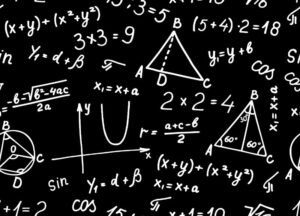## How do you solve a linear equation in algebra?

Solving a linear equation involves finding the value of one or both variables. This process is similar to adding and subtracting, which students are likely to be familiar with. The main difference is that a linear equation usually has only one or two variables, rather than a few. In addition, a linear equation has a degree that is always equal to 1.### How to solve a linear equation?

The basic principle of solving an equation is to isolate the variable, which means rewriting an equivalent equation in which the variable is on one side and everything else is on the other. This is done by dividing the number on one side of the equation by the number on the other side or multiplying or subtracting the two numbers.

In addition to the basic arithmetic operations, solving a linear equation involves using the elimination method. This eliminates any of the coefficients in the original equation so that the equation can be solved to find another equation.

### Graphing Linear Equations.

When solving a linear equation, students often begin by drawing points on a coordinate grid that correspond to the (x, y) pairs of values they have found in their equation. Then they plot all of the other points in their graph to make a straight line.

For some equations, this can be a simple task, but for others it may require some computation or a little bit of knowledge about how to graph lines. For example, when students are learning how to solve a two-step linear equation, they can draw the point (4,1), then the point (2,0), then the point (0,-1) and finally the point (-2, -2).

### Writing a Linear Equation.

Students can also write their linear equations in slope intercept form or in point slope form. Slope intercept form is an especially helpful technique for helping students visualize the equation because it makes the slope obvious. Slope intercept form is the equation y=mx+b, where m is the slope and b is the y-intercept.

If students have been comfortable with solving a two-step equation, they can try a more challenging method by creating a linear equation in slope intercept form. This involves rewriting the equation in slope intercept form and then calculating the slope and the y-intercept of the line.

Alternatively, they can try a more complicated method by converting the equation into standard form. This is a way to express a linear equation in which the constants, A, B, and C, must be integers and A must be positive.

Standard form is a useful tool for introducing the concept of linear equations to your students. It also helps them to understand why these equations are important.

In addition, standard form allows you to easily turn a linear equation into a system of equations containing two variables. This can be a very effective way to teach students the different ways that systems of equations can be solved.

How to Solve a Linear Equation Conclusion: Once students have learned how to solve a linear equation, they can apply their skills to other problems in algebra. For example, they can use their understanding of linear equations to help them understand a skateboard manufacturer’s costs and revenue when they introduce a new line of boards. They can also use their understanding of linear equations to help solve a problem about how many skateboards need to be sold before the manufacturer makes a profit.